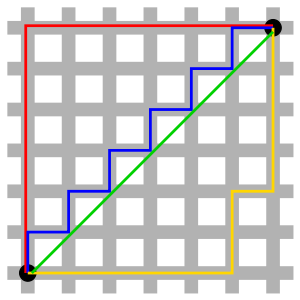# 机器学习中的数学——距离定义（二）：曼哈顿距离（Manhattan Distance）维空间中的欧几里得距离：``````def ManhattanDistance(x, y):
import numpy as np
x = np.array(x)
y = np.array(y)
return np.sum(np.abs(x-y))
``````

(0)